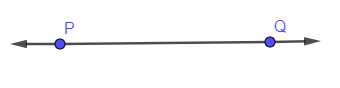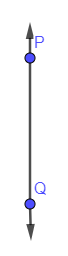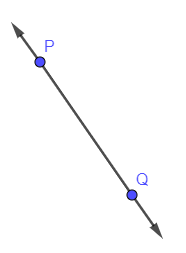Filters
Latest Questions
Mathematics
Construction of lines
Draw a line segment of length 6.3 cm. Draw another line parallel to it at a distance 3 cm from it.

Mathematics
Construction of lines
Draw a rough figure and label suitably in each of the following cases:
(a) Point P lies on the line segment AB.
(b) XY and PQ intersect at M.
(c) Line I contains E and F but not D.
(d) OP and OQ meet at O.

Mathematics
Construction of lines
Draw a line segment AB of 4 cm in length. Draw a line perpendicular to AB through A and B respectively. Are these lines parallel ?

Mathematics
Construction of lines
Draw a line, say AB take a point c outside it. Through C, draw a line parallel to AB using ruler and compasses only.

Mathematics
Construction of lines
Construct the parallel line with steps.

Mathematics
Construction of lines
Draw a line in your notebook and name it using a small letter of the alphabet.

Mathematics
Construction of lines
To draw parallel lines we use, \[\]
A. Compass \[\]
B. Divider \[\]
C. Scale and set squares \[\]
D. Scale and protractor \[\]

Mathematics
Construction of lines
Draw a line perpendicular to a line y. Take a point A on this perpendicular such that A is 4.6 cm away from y. Through A draw a line parallel to y.

Mathematics
Construction of lines
Draw a line AB of length 8 cm. At each end of this line segment, draw a line perpendicular to AB. Are these two lines parallel? State Yes or No.

Mathematics
Construction of lines
Construct a line parallel to the following lines from a point anywhere lying outside the lines. (a)(b)(c)Mathematics
Construction of lines
Draw a line CD parallel to the given line AB at a distance of 5.5 cm from it.
Mathematics
Construction of lines
Draw an angle of measure \({30^ \circ }\)and construct its bisector.

Prev
1
2